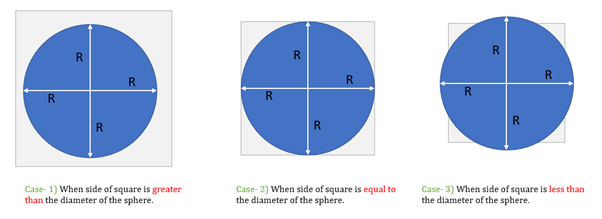# A noncompressible ball in the shape of a sphere is to be passed through a square opening in a board. – OG 2020 Question #331 with Solution

[two_third]

## OG 2020: Question No. 331

A noncompressible ball in the shape of a sphere is to be passed through a square opening in a board. What is the perimeter of the opening?

1. The radius of the ball is equal to 2 inches.
2. The square opening is the smallest square opening through which the ball will fit.

[/two_third]

[one_third_last]

 Source OG 2020 Type Data Sufficiency Topic Geometry Sub-Topic Rectangular Solids Difficulty Hard.

[/one_third_last]

### Solution

Steps 1 & 2: Understand Question and Draw Inferences

In this question, we are given

• A noncompressible ball in the shape of a sphere is to be passed through a square opening in a board.

We need to determine

• The perimeter of the opening.

As the sphere-shaped ball must pass through the square opening, there can be any of the three cases.Only in first two cases, sphere can pass through the square.

Hence, we can say

• The side of the square must either be equal to or greater than the diameter of the sphere for the sphere to pass.

With this understanding, let us now analyse the individual statements.

Step 3: Analyse Statement 1

As per the information given in statement 1, the radius of the ball is equal to 2 inches.

• However, from this statement, we do not have any information about the side of the square.

Hence, statement 1 is not sufficient to answer the question.

Step 4: Analyse Statement 2

As per the information given in statement 2, the square opening is the smallest square opening through which the ball will fit.

• However, from this statement, we have no information about the diameter of the ball, using which we can determine the perimeter of the square opening.

Hence, statement 2 is not sufficient to answer the question.

Step 5: Combine Both Statements Together (If Needed)

Combining information from both statements, we can say

• The diameter of the sphere ball is 4 inches.
• And the square opening is the smallest one through which the ball will fit.
• Therefore, the side of the square = 4 inches.
• And the perimeter of the opening = 4 * 4 inches = 16 inches

Thus, we can determine the answer by combining the information from both statements.

Hence, the correct answer is option C.Payal Tandon